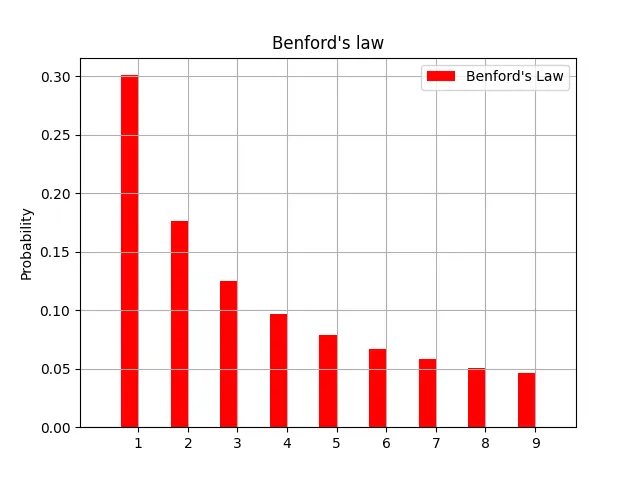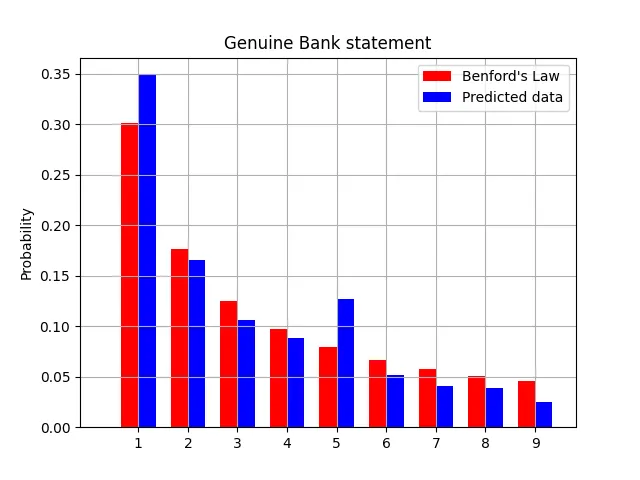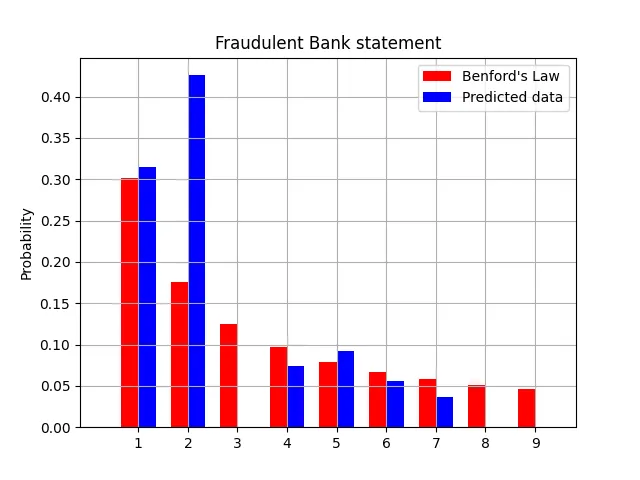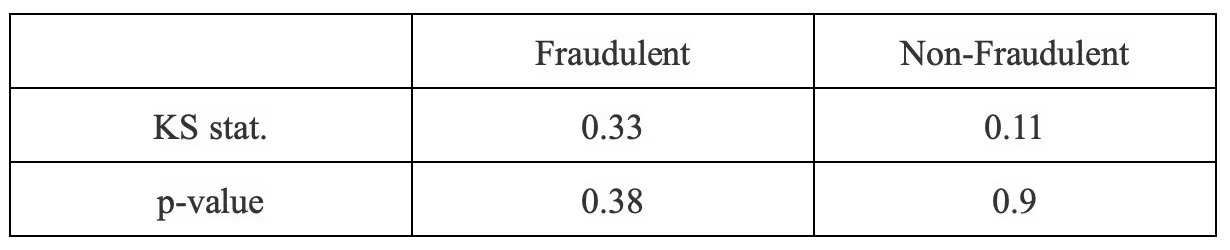# Bank Fraud and Benford’s Law

January 7, 2022

During the first half of the financial year 2021–22, the reported number of fraud cases in various banking operations increased to 4,071 as against 3,499 in the year-ago period, the RBI’s Report on Trend and Progress of Banking in India 2020–21 showed. - The Economic Times

A large number of these fraudsters are borrowers that tend to manipulate their investment in order to seek loans, what they fail to recognise is the power of probability distribution.

One may wonder — what does probability have to do with manipulating transactions, it’s just about adding random numbers as transactions isn’t it?

Frank Benford, a general electric physicist, may argue otherwise. As he, having observed close 20,000 numerical sets of data, generalized statistically the randomness of numbers. The statistical observation is popularly known as the law of first digits or Benford’s law.

The observation Benford made is — “In numerical datasets that obey the law, the number 1 appears as the leading significant digit about 30 % of the time, while 9 appears as the leading significant digit less than 5 % of the time. If the digits were distributed uniformly, they would each occur about 11.1 % of the time. Benford’s law also makes predictions about the distribution of second digits, third digits, digit combinations, and so on.”What the above graph signifies is that in a large, random set of numbers, the numbers leading with 1 e.g. 1, 12, 1434,195325 occur roughly 30% of the time. Similarly, numbers leading with 2 e.g. 27, 2048, 291 etc occur roughly 17% of the time and numbers leading with 9 e.g. 95, 911, 93942 etc. occur slightly less than 5% of the time.

There you have it, a simple statistical tool to flag anomalies and discrepancies in any numerical data. The same can be applied to any kind of numerical dataset, may it be electricity bills, street addresses, stock prices, house prices, population numbers, mortality rates, lengths of rivers and so on.

It’s funny how randomness isn’t random. Quite paradoxical, isn’t it?

Not convinced? We were somewhat in a pickle ourselves. So we set off to test out the law ourselves by taking two bank statements: a genuine and a manipulated one.In the above two graphs, the Red Bars represent Benford’s ideal Distribution. The Blue Bars represent the percentage distribution of the leading number while taking into account all amount values in the respective Bank Statement.

You may observe that the one on the right does not follow the distribution according to Benford’s law whereas the one on the left is pretty compliant with the same.

While Graphs are good for a visual representation, we would require to quantify the similarity between the patterns.

It is safe to assume that any deviations from Benford’s law in the data set would imply manipulation of numericals. To which we performed statistical tests via the Kalmogorov-Smirnov test (KS test) and their corresponding p-values. In the table below, we can see that the similarity between the Benford Distribution and Genuine Bank Statement distribution (as indicated by p-value) is 90%. The same for Fraudulent Bank Statement is 38%.Note: The Kolmogorov–Smirnov test can be modified to serve as a goodness of fit test. This test compares the underlying continuous distributions F(x) and G(x) of two independent samples. The p-value, or probability value, tells you how likely it is that your data could have occurred under the null hypothesis.

The same can be applied to a multitude of financial documents from budget reports to grocery bills, comforting financial organisations that a fraudster manipulating numeric data, no matter how random — will not escape Benford’s law.

One caveat to using Benford’s law is that the numbers have to be fairly large for the sample set to give accurate results.

Looking forward to test out more fraud detection methods in the future!